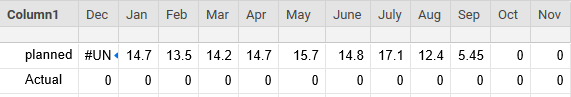# countif column values based on a monthly value

Hi,

I am creating a table of count of values in a column based on what month is reported in another. the table I want is attached. The formula I am using is as below. Can anyone advise what need to change in the planned formula

=SUMIF{V2.0 Eirabot - Gantt Range 1}},"12", {V2.0 Eirabot - Gantt Range 1}

I also want to have an actual row beneath. I have found issues where the two different calcs are providing the same values. The fact that smartsheets demotes a random range name rather than a pre-defined column name makes it difficult to reconsiliate. Any advice?

regards

AlanTags:

• If all you need is a count then try something like this...

=COUNTIFS({V2.0 Eirabot - Gantt Range 1}, MONTH(@cell) = 12)

.

In regards to your formula posted:

=SUMIF{V2.0 Eirabot - Gantt Range 1}},"12", {V2.0 Eirabot - Gantt Range 1}

You are missing an opening parenthesis, you have an additional curly bracket after the first cross sheet reference, you are not specifying that the MONTH needs to be 12 so the formula is looking for the EXACT text of "12", the second cross sheet reference being the same as the first means that you are trying to sum Range 1 which only needs to be specified if different from the criteria range (won't break anything by repeating it as long as proper data is in there, just means a few extra unnecessary steps), and there is no closing parenthesis.

To sum based on dates, you would need something like this...

=SUMIFS({Cross Sheet Reference Range to be Summed}, {Cross Sheet Reference Range containing Dates}, MONTH(@cell) = 12)

• thanks @paul

I tried the following for sumif

=SUMIFS({V2.0 Eirabot - Effort}, {V2.0 Eirabot - date}, MONTH(@cell) = 12)

does this look correct?

• Yes. That would be correct if you are trying to add values together based on the month in the date column being that of December.

• Hi Paul,

thanks for the continued support. I got the table of FTE's per month working with the following formula

=[SUMIF([V2.0 Eirabot - month1],12,{V2.0 Eirabot - Effort})]/160

• Excellent. Happy to help.## Help Article Resources

Want to practice working with formulas directly in Smartsheet?

Check out the Formula Handbook template!